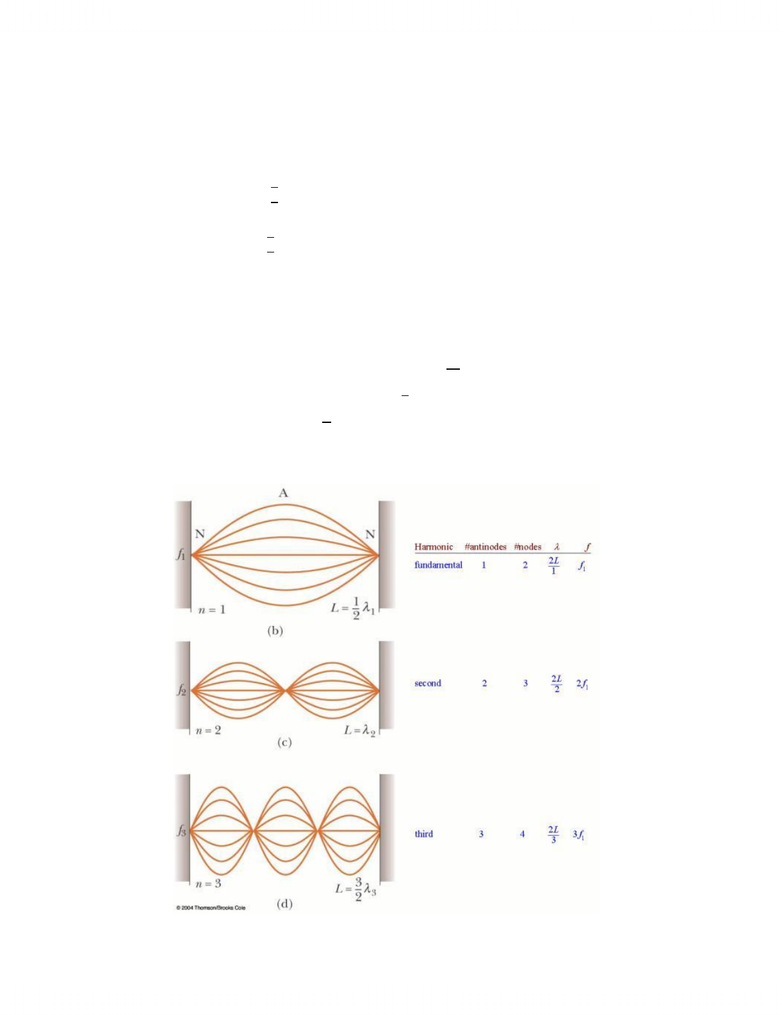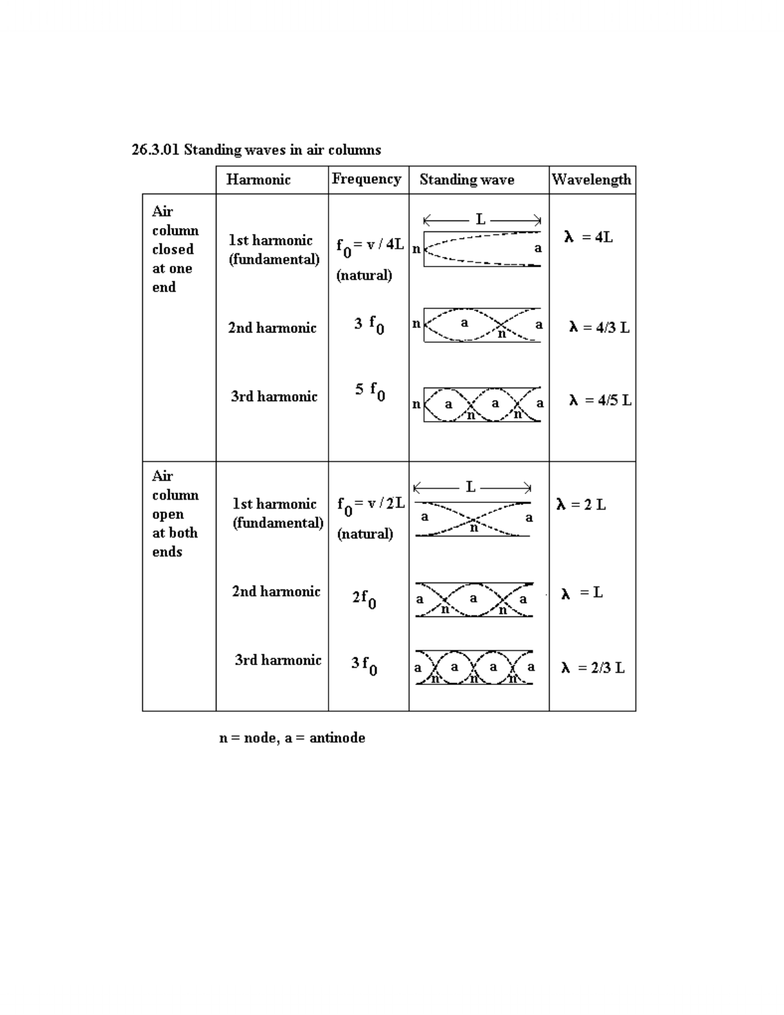Study Guides (380,000)
CA (150,000)
UW (6,000)
PHYS (60)
PHYS112 (10)

PHYS112 Study Guide - Charge Conservation, Superposition Principle, R V R

Department
Physics
Course Code
PHYS112
Professor
Carey Bissonnette

This preview shows pages 1-3. to view the full 9 pages of the document.PHYS 112 Review
Simple Harmonic Motion
- Non constant acceleration
- Periodic motion: motion that repeats itself
- Oscillatory motion: periodic motion that moves back and forth over the same path
Period (T): the time required for a motion to repeat, time required for one cycle of periodic motion
Frequency: the number of oscillations per unit time: f=1/T
Angular Frequency: w = 2πf = 2π/T
Position: x(t)=Acoswt
Velocity: v(t)=-Awsin(wt)
Acceleration: a(t)=-Aw2cos(wt) = -w2x(t)
Where A is the amplitude of motion, w is the angular frequency of motion, and f is the frequency of
motion
A linear restoring force will give you simple harmonic motion. The linear restoring force tries to push the
mass back to equilibrium position.
Hooke’s Law: F= -kx = ma
KE= ½mv2
PE= ½kx2
Energy doesn’t change when mass is doubled, however, maximum velocity does.
Chapter 14: Waves and Sound
Periodic disturbance transfer of energy without transferring materials
2 kinds of waves: transverse and longitudinal
Transverse: disturbance is perpendicular to direction of propagation
Longitudinal: disturbance is parallel to direction of propagation
v: velocity of wave (constant for constant medium)
A: amplitude (height of disturbance)
λ: wavelength, repeated disturbance
f: frequency, number of full waves that pass a point
T: period, time for one wavelength to pass a point
v=distance/time=x/T=fλ

Only pages 1-3 are available for preview. Some parts have been intentionally blurred.Superposition principle: 2 waves travelling toward each other meet and pass through each other. Where
the waves overlap you see the sum of the two waves:
constructive interference (waves that give a larger amplitude), in phase, louder noise, r1=r2+nλ
destructive interference (waves that give a smaller amplitude), out of phase, quiet noise r1=r2+(n+1/2 )λ
Sound: in solid medium:  
in fluid or gas medium:  
When a sound wave passes from air to water, wave speed must change (different medium). However,
frequency does not change, as it is determined by the source. Now v= fλ, and because f is constant, and
v changed, λ must also change.
Sound source emits energy over time: Power of source  

Sound intensity (power passing through an area):  
Intensity level (sound level):  
, where I0 is the threshold of hearing, and β is in decibels.
Standing waves on a String

Only pages 1-3 are available for preview. Some parts have been intentionally blurred.Standing Waves in an Air Column